# KS1 Maths Quiz - Year 2 Money - Value of Coins (Questions)

This quiz addresses the requirements of the National Curriculum KS1 Maths and Numeracy for children aged 6 and 7 in year 2. Specifically this quiz is aimed at the section dealing with recognising coin values and finding different combinations of coins that equal the same amounts of money.

Children in Year 2 should be familiar with all the coins we use and their values. They should also recognise the values of different combinations, for example, that two £1 coins have the same value as a £2 coin or that two 50p coins have the same value as a £1 coin.

This quiz will help children to work out the values of different coin combinations.

To see a larger image, click on the picture.
1. What is the value of one of these coins?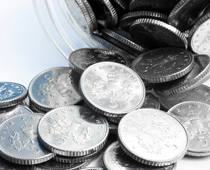[ ] £1 [ ] 20p [ ] 10p [ ] 5p
2. What is the value of one of these coins?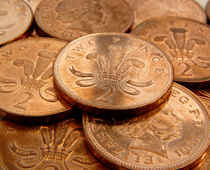[ ] 2p [ ] 1p [ ] 5p [ ] 10p
3. If I had 5 of these coins, I would have the same value as a...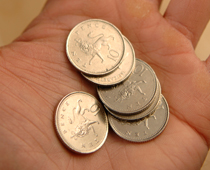[ ] 20p piece [ ] £1 coin [ ] 50p piece [ ] £2 coin
4. This coin is worth the same as...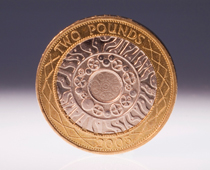[ ] two 50p pieces [ ] two £1 coins [ ] ten 5p pieces [ ] six 2p pieces
5. Together these coins have the same value as...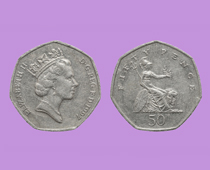[ ] 10p [ ] 20p [ ] £1 [ ] £2
6. Which coin here has the lowest value?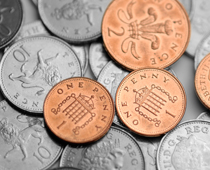[ ] 1p [ ] 2p [ ] 10p [ ] They all have the same value
7. How many 2p coins would have the same value as 20p?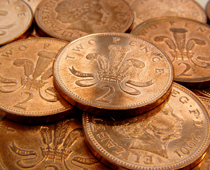[ ] 20 [ ] 10 [ ] 2 [ ] 5
8. How many of these coins would have the same value as £1?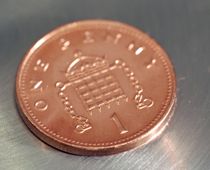[ ] 100 [ ] 10 [ ] 50 [ ] 20
9. What is the highest value coin in this handful?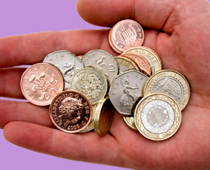[ ] £1 [ ] 50p [ ] 20p [ ] £2
10. Which coin is missing?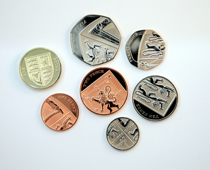[ ] £1 [ ] £2 [ ] 50p [ ] 2pKS1 Maths Quiz - Year 2 Money - Value of Coins (Answers)
1. What is the value of one of these coins?[ ] £1 [ ] 20p [ ] 10p [x] 5p
5p coins are much smaller than 20p and 10p pieces
2. What is the value of one of these coins?[x] 2p [ ] 1p [ ] 5p [ ] 10p
A 2p coin has the same value as two 1p coins
3. If I had 5 of these coins, I would have the same value as a...[ ] 20p piece [ ] £1 coin [x] 50p piece [ ] £2 coin
Five ten pence pieces has the same value as one 50p piece
4. This coin is worth the same as...[ ] two 50p pieces [x] two £1 coins [ ] ten 5p pieces [ ] six 2p pieces
This is a £2 coin, worth 2 x £1
5. Together these coins have the same value as...[ ] 10p [ ] 20p [x] £1 [ ] £2
2 x 50p = 100p and 100p = £1
6. Which coin here has the lowest value?[x] 1p [ ] 2p [ ] 10p [ ] They all have the same value
The 1p is the lowest value coin we use
7. How many 2p coins would have the same value as 20p?[ ] 20 [x] 10 [ ] 2 [ ] 5
10 x 2 = 20
8. How many of these coins would have the same value as £1?[x] 100 [ ] 10 [ ] 50 [ ] 20
There are 100 pennies in a pound
9. What is the highest value coin in this handful?[ ] £1 [ ] 50p [ ] 20p [x] £2
The £2 coin is the highest value coin we have
10. Which coin is missing?[ ] £1 [x] £2 [ ] 50p [ ] 2p
We have 8 different coins altogether